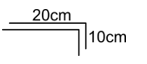Methods to locate centre of mass
Question

# A rope of length 30 cm is on a horizontal rough table with maximum length hanging from edge ‘A’ of the table. The coefficient of friction between rope and table is 0.5. The distance of centre  of mass of the rope from ‘A’ is

Difficult
Solution

##$\mathrm{\mu }\left(\mathrm{l}-\frac{\mathrm{l}}{\mathrm{n}}\right)\mathrm{mg}=\mathrm{mg}\frac{\mathrm{l}}{\mathrm{n}}$$\mathrm{\mu }\left(1-\frac{1}{\mathrm{n}}\right)=\frac{1}{\mathrm{n}}$$0.5\left(\frac{\mathrm{n}-1}{\mathrm{n}}\right)=\frac{1}{\mathrm{n}}$$\mathrm{n}-1=2;\text{\hspace{0.17em}\hspace{0.17em}\hspace{0.17em}\hspace{0.17em}\hspace{0.17em}\hspace{0.17em}\hspace{0.17em}\hspace{0.17em}\hspace{0.17em}\hspace{0.17em}}\mathrm{n}=3$The total mass is m .The length of the rope hanging is  $\frac{\mathrm{I}}{3}$ and the length of the rope on the table is $\frac{2\mathrm{I}}{3}$  whose mass is equal to$\frac{2\mathrm{m}}{3}\to {\mathrm{r}}_{\mathrm{cm}}\frac{\frac{2\mathrm{m}}{3}\left(-10\right)\stackrel{^}{\mathrm{i}}+\frac{\mathrm{m}}{3}\left(-5\stackrel{^}{\mathrm{j}}\right)}{\mathrm{m}}=\frac{-20\stackrel{^}{\mathrm{i}}-5\stackrel{^}{\mathrm{j}}}{3}$$\left|{\mathrm{r}}_{\mathrm{cm}}\right|=\sqrt{\frac{400}{9}+\frac{25}{9}}=\sqrt{\frac{425}{9}}=\frac{5\sqrt{17}}{3}$

Get Instant Solutions